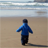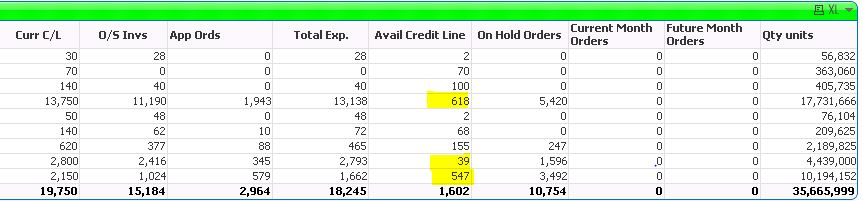# QlikView App Dev

Discussion Board for collaboration related to QlikView App Development.

Announcements
On May 18th at 10AM EDT we will answer your QlikView questions live. REGISTER
cancel
Showing results for
Did you mean:Creator II

## Problem with expression in a straight table some figures incorrect

I have this calculation in my straight table, all rows are calculating the  correct figure except three rows highlightedMy expression is this for avail credit line column, I have also attached the settings from chart properties.

(Sum([Credit Limit])

-

((Sum({<[OEL On Hold]={'Y'}, [OEL Credit Hold]={'N'}, OE_Order_Lines_All.INVOICE_INTERFACE_STATUS_CODE-={'YES'}>

+

<[OEL On Hold]={'N'}, OE_Order_Lines_All.INVOICE_INTERFACE_STATUS_CODE-={'YES'}>}

If([Line Calc Tax Rate]>0,

OE_Order_Lines_All.ORDERED_QUANTITY * OE_Order_Lines_All.UNIT_SELLING_PRICE*((100+[Line Calc Tax Rate])/100),

OE_Order_Lines_All.ORDERED_QUANTITY * OE_Order_Lines_All.UNIT_SELLING_PRICE)

)

+

Sum({<[OEL On Hold]={'Y'}, [OEL Credit Hold]={'N'}, OE_Order_Lines_All.INVOICE_INTERFACE_STATUS_CODE-={'YES'}>

+

<[OEL On Hold]={'N'}, OE_Order_Lines_All.INVOICE_INTERFACE_STATUS_CODE-={'YES'}>}

[Freight Amount])

)

+

(Sum([Acctd Amount Due Remaining])-Sum(Alt(AR_Receivable_Applications_All.AMOUNT_APPLIED,0)))))/1000

2 RepliesMVP & Luminary

Try it with a range-function for adding the various expression-parts, like:

rangesum(sum1, sum2, sum3, -sum4)

- MarcusCreator II
Author

Thank you Marcus, I figured out why it wasn't working for the highlighted figures, I was missing some transactions from another table. Thank you again for your help though.

regards

Mina